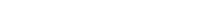# Math riddles Level 48 Answer with Hints and solutionsMath riddles game level 48 Answer with solution android game developed by Black games. Scroll Below to find details.

Math Riddles tests your IQ with mathematical puzzles. Challenge yourself with different levels of math puzzles and stretch the limits of your intelligence. Every IQ game is prepared with an approach of an IQ test. Improve your mathematics, challenge your brain with this game. There are many hard levels which you may not have solved then you can take help from here.

Math app level 48:Solution:

42=(3+1)(3-1)

82=(5+3)(5-3)

similarly

53=(4+1)(4-1)

If there is any Doubt/discrepancy then please let me know in comments or you are unable to understand solution then I will surely help you.

### 5 thoughts on “Math Riddles App Level 48 Solution Android”

•May 19, 2019 at 8:04 pm

3 42 1 42: (3+1)×10 +(3-1)
5 82 3 82: (5+3)×10 +(5-3)
6 84 2 84: (6+2)×10 +(6-2)
4 ?? 1 ??: (4+1)×10 +(4-1) = 53

•February 12, 2019 at 12:58 pm

i dident anderstand

•February 13, 2019 at 1:07 am

this is not multiplication, First digit is sum of two digit and second digit is Subtraction of two digits

•May 26, 2019 at 4:53 pm
•